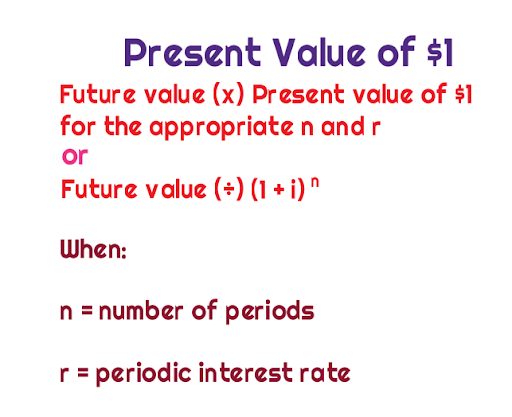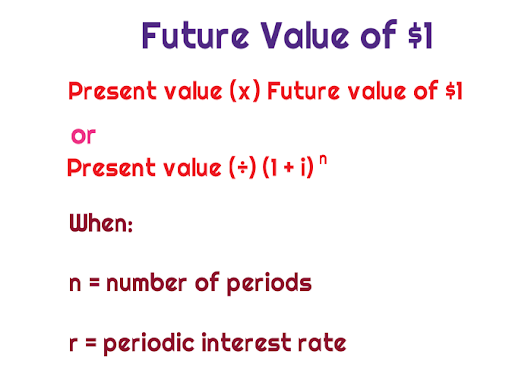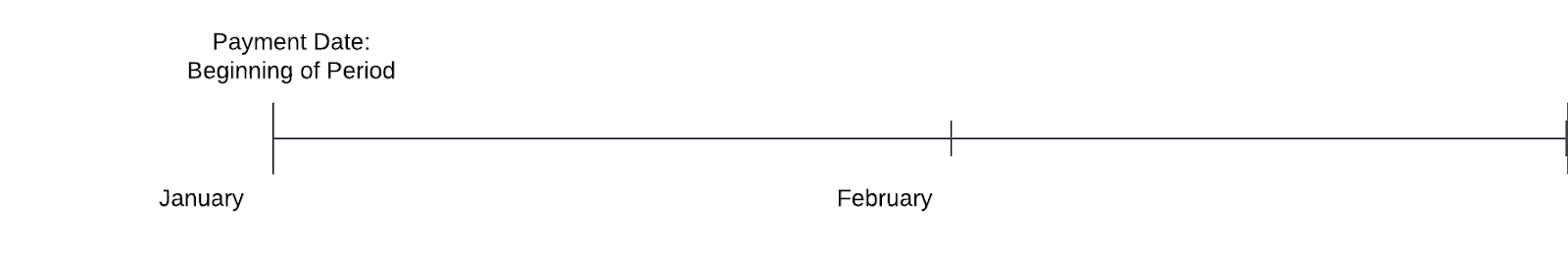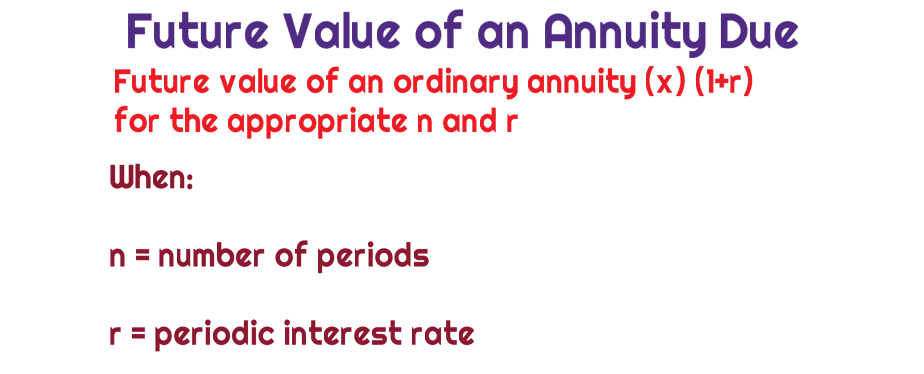The time value of money is the concept that money that is available at the present time will be worth more than that same amount in the future because of its potential earning capacity. For example, money that is deposited into a savings account will earn interest, and it will therefore be considered to be compounding in value. This is a concept that is extremely important and even tested on the most advanced business licensing exams such as the CFA and CPA exam. In this article we are going to break out the present value of \$1, the future value of 1, The present value of an ordinary annuity, the future value of an ordinary annuity, and the present value of an annuity due.The present value of \$1:

The present value of \$1 table dictates the present value discount rates that are used for various combinations of interest rates as well as time periods. The present value of \$1’s discount rate is selected from a table that is then multiplied by a cash sum that will be received at a future date. This will allow the arrival of its present value. In addition, the interest rate selected in the table can be based on the specific circumstances of the debt.If the present value of \$1 factor is not supplied in a test question, you will generally always be able to apply a supporting table to determine which factor to use.

The future value of \$1:

The future value of \$1 is considered the amount that would be determined at a future point in time if \$1 was invested today. This can also be known as compounded interest, and the interest factor will cause the future value to be greater than today’s value.If the future value of \$1 factor is not supplied in a test question, you will generally always be able to apply a supporting table to determine which factor to use.

The present value of an ordinary annuity:

The present value of an ordinary annuity is the present-day value of a series of payments to be made at the end of a period. The following is the formula to compute the present value of an ordinary annuity.If the present value of an ordinary annuity is not supplied in a test question, you will generally always be able to apply a supporting table to determine which factor to use.

The future value of an ordinary annuity:

The future value of an ordinary annuity is a series of lump payments that occur at a specified point in time and will be derived in the same amounts. The future value of an ordinary annuity is the value at the end of a period. The following is the calculation for an ordinary annuity.If the future value of an ordinary annuity is not supplied in a test question, you will generally always be able to apply a supporting table to determine which factor to use.

The present value of an annuity due:

“The payment is DUE today”. The present value of an annuity due is considered the same concept as the present value of an ordinary annuity, however, in an annuity due a single payment made at the beginning of the period.If the present value of an annuity due is not supplied in a test question, you will generally always be able to apply a supporting table to determine which factor to use.

The future value of an annuity due – “The payment is DUE today”. The future value of an annuity due is considered the same concept as the future value of an ordinary annuity, however, in an annuity due a single payment made at the beginning of the period.If the future value of an annuity due is not supplied in a test question, you will generally always be able to apply a supporting table to determine which factor to use.

Recap:

At the end of the day, the most important takeaway is for you to focus on when the payment is being made (e.g., beginning or end of the period). If you are a visual learner, hopefully this clarifies a lot of your understanding. I think we are all visual learners, and by seeing it, it should be easier for you to imprint this in your brains.Author Bio:
Joey Reeve is the founder and CEO of Universal CPA Review, a New York City based EdTech company. Universal is known for its application of visual learning techniques and adaptive learning technologies in helping candidates pass the CPA exam. He is responsible for overseeing product development and go-to-market strategies. You can find Joey on LinkedIn or by visiting Universal CPA Review’s website.

Article posted in Personal Finance

Tagged

This site uses Akismet to reduce spam. Learn how your comment data is processed.